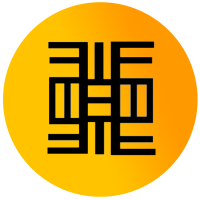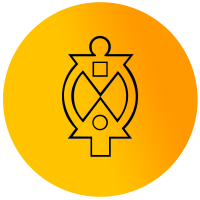# Best 1:1 Private Online & In-home Pre-Calculus Math Tutors Near MeDOORSTEP & ONLINE SERVICES

• New York
• NYC (New York City)
• Manhattan
• Brooklyn
• Queens
• Staten Island
• Bronx
• Long Island including the Hamptons
• Westchester
• New Jersey
• Connecticut
• and remote to any location!

CHECK US OUT

DOORSTEP & ONLINE SERVICES

• New York
• NYC (New York City)
• Manhattan
• Brooklyn
• Queens
• Staten Island
• Bronx
• Long Island including the Hamptons
• Westchester
• New Jersey
• Connecticut
• and remote to any location!

CHECK US OUT

HELPFUL LINKSRATES

MEET OUR FOUNDER AND STAFF

THEMBA AND THE COMPETITION

FINDING A QUALIFIED TUTOR

STAFF’S BIOS

SCHOOLS WE WORK WITH

TESTIMONIALS## PRE-CALCULUS

What Is Pre-Calculus?

Pre-calculus is studying the mathematical prerequisites for calculus, including algebra, trigonometry, and analytic geometry. The unusual thing about pre-calculus topics is that they don’t directly involve calculus. Instead, they give students a strong foundation that will be used throughout their calculus studies.

PRECALCULUS FINAL EXAM REVIEW

Looking for a pre-calculus tutoring service? Contact us!Pre-Calculus and Trigonometry

Trigonometry is the study of the relationships between the dimensions and angles of triangles. It is a full course by itself in most high school and college math departments, so pre-calculus coverage mostly serves as a refresher. Taking a trigonometry course is often required before enrolling in pre-calculus. During pre-calculus, you can expect to solve and graph problems using standard trigonometry functions like sine and cosine. Additional topics covered in pre-calculus include vector operations, sequences, and series.

Pre-Calculus and Algebra

Many educators say that strong algebra skills are the key to success in calculus. In addition to reviewing the trigonometric functions, pre-calculus covers algebraic functions such as quadratic, exponential, polynomial, and logarithmic. The graphing of functions is an important part of pre-calculus since graphs are used throughout calculus. The domain and range of functions are covered, along with finding the intervals over which a function increases or decreases and performing transformations on a function.

## Is Pre-Calculus Difficult to Learn?

A pre-calculus course in high school is not difficult. It is a more tedious version of Algebra and where you deal with complex fractions and decimals and do more advanced factoring. You will learn new topics such as the long division of polynomials and the remainder theorem and limits.

What Are the Prerequisites for Pre-Calculus?

To take a pre-calculus class, students need to have studied the following concepts and skills that are divided into six categories.

RENAMING EXPRESSIONS

Base ten number system; arithmetic with decimals, fractions, signed numbers; set notation (interval, set-builder, union, intersection); basic vocabulary (e.g., the phrases “at least” and “at most,” nonnegative, integers, consecutive); percentages; unit conversion; scientific notation; factoring; radicals; exponent laws; polynomials; matrices; complex numbers; completing the square technique; long division of polynomials; and logarithms.

SOLVING EQUATIONS AND INEQUALITIES IN ONE VARIABLE

Linear; quadratic; absolute value; exponential; logarithmic; radical; systems; rational; compound inequalities; and the zero factor law. Understand extraneous solutions and when they can arise. Be sure that you can distinguish between exact and approximate solutions. You should understand the relationship between the algebraic and graphical solutions of sentences.

GRAPHING SENTENCES IN TWO VARIABLES

Familiarity with these “basic models”: y = x , y = x 2 , y = x 3 , y = |x| , y = √ x , y = 1 x , y = k , y = ln x (and other bases) , y = ex (and other bases). Be able to graph circles and lines. Be able to graph transformations of the “basic models” involving: horizontal and vertical translations, vertical scaling; reflection about the x-axis; absolute value transformation. Be able to handle compound sentences that use the mathematical words ‘and’ and ‘or.’

Looking for pre-calculus tutoring services? Contact us!BASIC GEOMETRY FORMULAS

Perimeters of common figures, including the circumference of a circle. Also, know the following formulas: AREA: rectangle, triangle, circle, trapezoid VOLUME: right cylinder (with familiar base)

FUNCTIONS

Function notation; domain and range; composition; piecewise-defined functions; quadratic (y = ax2 +bx+c and y = a(x−h) 2 +k forms); higher-order polynomial (relationship between the zeros and factors); exponential and logarithmic (allowable bases, shapes of graphs); rational (asymptotes, end behavior, puncture points); and periodic (sine and cosine).

CALCULATOR SKILLS

Key expressions using correct knowledge of the order of operations; store and recall named variables; use stored values in calculations. Graph functions: set the window; trace along a curve; find maxima/minima of graphs; find x-intercepts using the built-in calculator feature; use the table feature; use the Zoom In, Zoom Out, and Z-Box features; and find intersection points of graphs.

How Can Themba Tutors Help?

Many students find math frustrating or confusing. If your student struggles to keep up with the pre-calculus curriculum, then mastering new material can seem like an impossible task. Plus, when new concepts are introduced upon previous material, falling behind can lead to issues down the road.

Perhaps this explains why qualified, engaging, and encouraging math tutors are some of the most sought after professionals when it comes to after school support. A private math tutor creates a safe space where students can learn new concepts at their own pace. Pre-calculus tutoring allows students to explore concepts, ask questions, and receive positive reinforcement. Ultimately, working with tutors enables students to gain mastery of pre-calculus and confidence and a sense of personal accomplishment as their grades improve.

Our pre-calculus tutoring focuses on two key areas. First, we look to fill any gaps so students have a strong foundation for the building. Second, we work to help students learn and master the new concepts that arise each week. The goal is to ensure that they have mastered the material and are prepared for tests, quizzes, and other assignments. Themba Tutors aims to maximize their learning potential from a collaborative approach working with parents, teachers, and other professionals.

## FREQUENTLY ASKED QUESTIONS

##### Is Pre-Calculus hard?

Precalculus, which is a combination of trigonometry and math analysis, bridges the gap to calculus, but it can feel like a potpourri of concepts at times. Students are suddenly required to memorize a lot of material as well as recall various concepts from their previous math courses.At Themba Tutors, we will let you know what kind of help you need once we set up a free intake. Knowing the student’s strengths and weaknesses is vital for helping the student with the challenges they’re facing. We offer services that include academic coaching and tutoring based on the student’s needs. We also offer a hybrid model for those students who need help with content and improving their underlying study skills.

HELPFUL LINKSRATES

MEET OUR FOUNDER AND STAFF

THEMBA AND THE COMPETITION

FINDING A QUALIFIED TUTOR

STAFF’S BIOS

SCHOOLS WE WORK WITH

TESTIMONIALSContact Themba Tutors Today!

Our Pre-calculus tutoring program is customized for all skills levels!

Call: (917) 382-8641, Text: (201) 831-9848

Email: info@thembatutors.com

(we respond to email right away!).